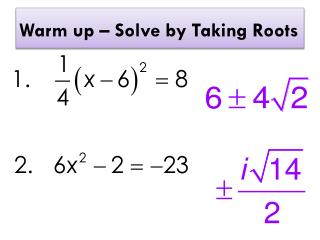DownloadDownload PresentationWarm up – Solve by Taking Roots

# Warm up – Solve by Taking Roots

Télécharger la présentation## Warm up – Solve by Taking Roots

- - - - - - - - - - - - - - - - - - - - - - - - - - - E N D - - - - - - - - - - - - - - - - - - - - - - - - - - -
##### Presentation Transcript

1. Warm up – Solve by Taking Roots

2. Questions over HW?

3. The Discriminant

4. Put the quadratic in standard form first!

5.  If the discriminant is POSITIVE, then you will have 2 real roots.  If the discriminant is ZERO, then you will have 1 real root.  If the discriminant is NEGATIVE, then you will have 2 imaginary roots.

6. Discriminant Foldable Notes Fold your paper over (top to bottom leaving about an inch at the bottom). Positive ZerO Negative

7. Discriminant 2 real Roots ZerO Negative

8. Discriminant One real Root Positive Negative

9. Discriminant 2 imaginary Roots Positive ZerO

10. Determine the number & types of roots.Example: 1 2 Imaginary Roots

11. Determine the number & type of roots.Example: 3 2 Real Roots

12. Solving by the Quadratic Formula

13. Quadratic Formula Everything must be on the same side of the equals sign before solving

14. Question #1Use the quadratic formula to find the zeros.

15. Question #2Use the quadratic formula to find the zeros.

16. Question #3Use the quadratic formula to find the zeros.

17. Question #4Use the quadratic formula to find the zeros.